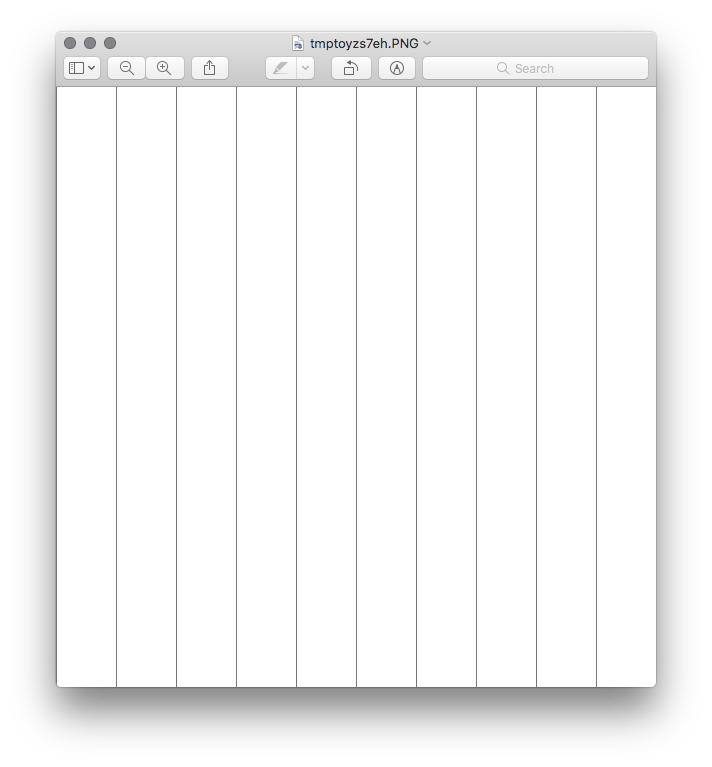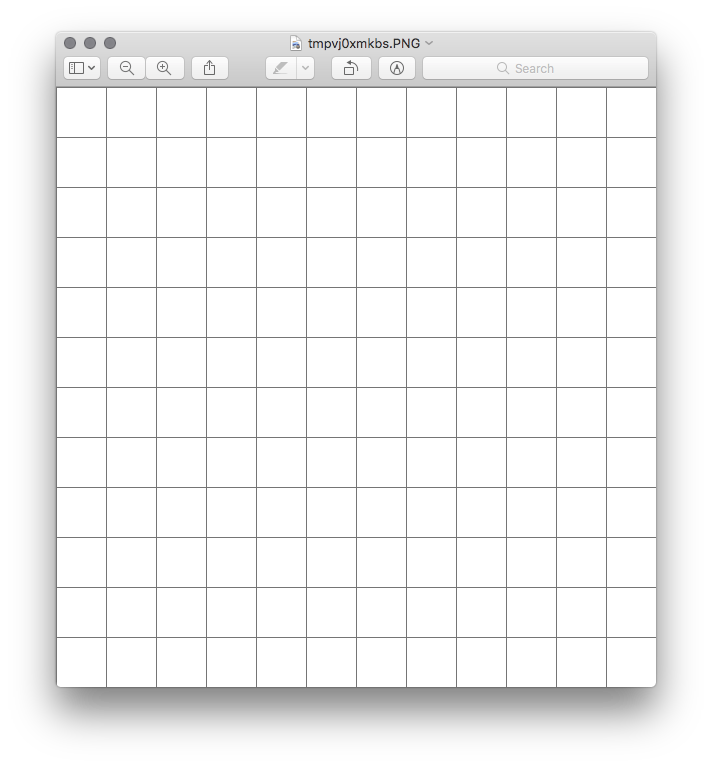Knotwork Practice
Friday, 15 December, 2017
My Ski Beanie
Sunday, 19 November, 2017

# Drawing Grids With Python and Pillow

Posted
post #python #Programming
Got a comment? A question? More of a comment than a question? Talk to me about this page on: twitter

Hey I used Python and Pillow to make grids for my drawing. Read on to watch my brain while I figured it out. Apologies for the minimal editing and the ridiculous number of images.

I draw. See? Many of my sketches have repeated elements, like zentangle or celtic inspired patterns. Okay, I don’t have many examples on the site. Sure there’s plenty of repetition based on symmetry tools in the drawing apps I use, and a little bit taking advantage of perspective grids. Not much in the way of simple grid-based repetition though.

Templates exist, but I want custom templates to fit the size of my workspace. I started exploring the Pillow library recently, so let’s use that to make custom grids for my drawings.

``````from PIL import Image

if __name__ == '__main__':
height = 600
width = 600
image = Image.new(mode='L', size=(height, width), color=255)

image.show()
``````

I use a modest 600 by 600 pixel grayscale image while working out the details. No point saving anything until I know what’s going on, so just `show()` the image.

Most of what I want is in the ImageDraw module.

## Simple Grid

``````from PIL import Image, ImageDraw

if __name__ == '__main__':
height = 600
width = 600
image = Image.new(mode='L', size=(height, width), color=255)

# Draw a line
draw = ImageDraw.Draw(image)
x = image.width / 2
y_start = 0
y_end = image.height
line = ((x, y_start), (x, y_end))
draw.line(line, fill=128)
del draw

image.show()
``````

Nice. Okay, how about repeating some lines across?

``````from PIL import Image, ImageDraw

if __name__ == '__main__':
height = 600
width = 600
image = Image.new(mode='L', size=(height, width), color=255)

# Draw some lines
draw = ImageDraw.Draw(image)
y_start = 0
y_end = image.height
step_size = int(image.width / 10)

for x in range(0, image.width, step_size):
line = ((x, y_start), (x, y_end))
draw.line(line, fill=128)

del draw

image.show()
``````Drawing some columns Lovely. How about an actual grid?

``````from PIL import Image, ImageDraw

if __name__ == '__main__':
height = 600
width = 600
image = Image.new(mode='L', size=(height, width), color=255)

# Draw some lines
draw = ImageDraw.Draw(image)
y_start = 0
y_end = image.height
step_size = int(image.width / 10)

for x in range(0, image.width, step_size):
line = ((x, y_start), (x, y_end))
draw.line(line, fill=128)

x_start = 0
x_end = image.width

for y in range(0, image.height, step_size):
line = ((x_start, y), (x_end, y))
draw.line(line, fill=128)

del draw

image.show()
``````

Okay cool but I often need a specific number of squares in my grid.

``````from PIL import Image, ImageDraw

if __name__ == '__main__':
step_count = 25
height = 600
width = 600
image = Image.new(mode='L', size=(height, width), color=255)

# Draw some lines
draw = ImageDraw.Draw(image)
y_start = 0
y_end = image.height
step_size = int(image.width / step_count)

for x in range(0, image.width, step_size):
line = ((x, y_start), (x, y_end))
draw.line(line, fill=128)

x_start = 0
x_end = image.width

for y in range(0, image.height, step_size):
line = ((x_start, y), (x_end, y))
draw.line(line, fill=128)

del draw

image.show()
``````

Right but I don’t want to edit the code every time.

``````import sys

from PIL import Image, ImageDraw

if __name__ == '__main__':
step_count = 10

if len(sys.argv) == 2:
step_count = int(sys.argv)

height = 600
width = 600
image = Image.new(mode='L', size=(height, width), color=255)

# Draw some lines
draw = ImageDraw.Draw(image)
y_start = 0
y_end = image.height
step_size = int(image.width / step_count)

for x in range(0, image.width, step_size):
line = ((x, y_start), (x, y_end))
draw.line(line, fill=128)

x_start = 0
x_end = image.width

for y in range(0, image.height, step_size):
line = ((x_start, y), (x_end, y))
draw.line(line, fill=128)

del draw

image.show()
``````

Run it.

``````\$ python grid.py 12
``````Grabbing a step count from the command line

I can specify step count from the command line. Cool. Uh hey about height and width?

``````import sys

from PIL import Image, ImageDraw

if __name__ == '__main__':
step_count = 10
height = 600
width = 600

if len(sys.argv) == 2:
step_count = int(sys.argv)
elif len(sys.argv) == 3:
width = int(sys.argv)
height = int(sys.argv)
elif len(sys.argv) == 4:
width = int(sys.argv)
height = int(sys.argv)
step_count = int(sys.argv)

image = Image.new(mode='L', size=(height, width), color=255)

# Draw some lines
draw = ImageDraw.Draw(image)
y_start = 0
y_end = image.height
step_size = int(image.width / step_count)

for x in range(0, image.width, step_size):
line = ((x, y_start), (x, y_end))
draw.line(line, fill=128)

x_start = 0
x_end = image.width

for y in range(0, image.height, step_size):
line = ((x_start, y), (x_end, y))
draw.line(line, fill=128)

del draw

image.show()
``````

Oh come on. Stop it with `sys.argv`. Get some real command line handling in there.

``````import argparse

from PIL import Image, ImageDraw

if __name__ == '__main__':
parser = argparse.ArgumentParser()
parser.add_argument("width", help="width of image in pixels",
type=int)
parser.add_argument("height", help="height of image in pixels",
type=int)
parser.add_argument("step_count", help="how many steps across the grid",
type=int)
args = parser.parse_args()

step_count = args.step_count
height = args.height
width = args.width

image = Image.new(mode='L', size=(height, width), color=255)

# Draw some lines
draw = ImageDraw.Draw(image)
y_start = 0
y_end = image.height
step_size = int(image.width / step_count)

for x in range(0, image.width, step_size):
line = ((x, y_start), (x, y_end))
draw.line(line, fill=128)

x_start = 0
x_end = image.width

for y in range(0, image.height, step_size):
line = ((x_start, y), (x_end, y))
draw.line(line, fill=128)

del draw

image.show()
``````

Much better. Run it.

``````\$ python grid.py
usage: grid.py [-h] width height step_count

positional arguments:
width       width of image in pixels
height      height of image in pixels
step_count  how many steps across the grid

optional arguments:
-h, --help  show this help message and exit

\$ python grid.py 500 500 20
``````

I like Argparse.

Anyways - what if I ask for a rectangle instead of a square?

``````\$ python grid.py 400 600 24 \{\{< /console >}}
``````

image::grid-rectangular.png[Rectangular grid,title=“Rectangular grid”]

Hold on. I was handing `height` and `width` to Image in the wrong order this whole time.

``````if __name__ == '__main__':
# ...

image = Image.new(mode='L', size=(width, height), color=255)

# ...
``````

Run it.

``````\$ python grid.py 400 600 24
``````

This works. I have half a dozen ideas left, but I want to use it for a sketch now.

`grid.py`

``````import argparse

from PIL import Image, ImageDraw

if __name__ == '__main__':
parser = argparse.ArgumentParser()
parser.add_argument("width", help="width of image in pixels",
type=int)
parser.add_argument("height", help="height of image in pixels",
type=int)
parser.add_argument("step_count", help="how many steps across the grid",
type=int)
args = parser.parse_args()

step_count = args.step_count
height = args.height
width = args.width
image = Image.new(mode='L', size=(width, height), color=255)

# Draw a grid
draw = ImageDraw.Draw(image)
y_start = 0
y_end = image.height
step_size = int(image.width / step_count)

for x in range(0, image.width, step_size):
line = ((x, y_start), (x, y_end))
draw.line(line, fill=128)

x_start = 0
x_end = image.width

for y in range(0, image.height, step_size):
line = ((x_start, y), (x_end, y))
draw.line(line, fill=128)

del draw

filename = "grid-{}-{}-{}.png".format(width, height, step_count)
print("Saving {}".format(filename))
image.save(filename)
``````
``````\$ python grid.py 1800 2400 50
Saving grid-1800-2400-50.png
\$ ls
grid-1800-2400-50.png   grid.py*
``````

Let’s skim over the part where I get the grid onto the iPad and import it as a new layer in my current sketch. That part includes no code — for now.

Anyways, back to the sketch.